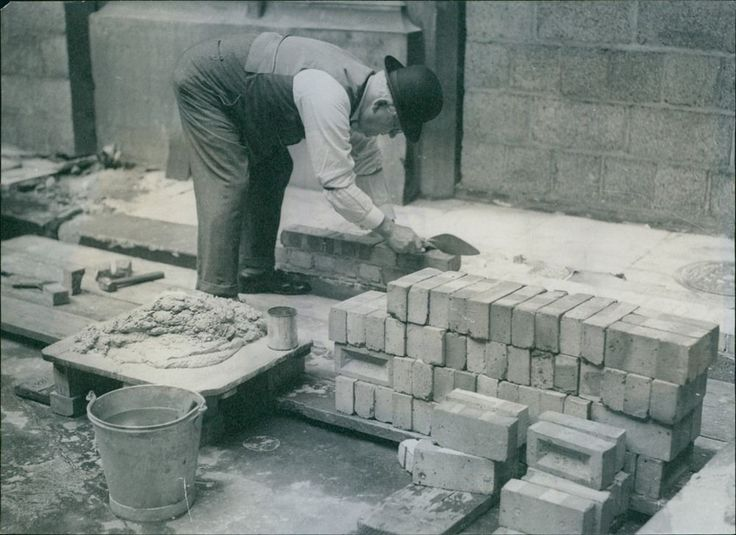# The Contractor's Problem

Calculus Level 3

A masonry contractor notes that for 1000 bricks, he needs 8 bags of mortar.For another 2000 bricks, he needs 16 bags of mortar. For yet another 3000 bricks, he needs 24 bags of mortar. He writes the fraction, where in the denominator $1=1000$

$\dfrac{8+16+24+32+40+48+\cdots}{1+2+3+4+5+6+\cdots}=8$

and so he reasons that he needs 8 bags of mortar for every 1000 bricks used. Is he right? Remember, he is also a mathematician.

×